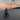# Clojure Naive Bayes under 100 LOC

October 09, 2020

Recently I was learning more about Bayes algorithms and how to implement a simple spam filter.

I wanted to have a simple and small implementation to help solidify the understanding of it.

I found an implementation in JS and decided to port it, the result is the following:

``````(ns com.wsscode.bayes
"Simple naive bayes implementation in Clojure.

Implementation ported from: https://github.com/ttezel/bayes/blob/master/lib/naive_bayes.js"
(:require [clojure.string :as str]))

(defn- math-log [n] #?(:clj (Math/log n) :cljs (js/Math.log n)))

(defn tokenize [s]
(into []
(comp (map str/lower-case)
(remove #(re-find #"^\d+\$" %)))
(str/split s #"[.\s,]+")))

(defn classifier []
{::vocabulary           #{}
::vocabulary-size      0
::total-documents      0
::doc-count            {}
::word-count           {}
::word-frequency-count {}
::categories           #{}})

(defn initialize-category
[{::keys [categories] :as classifier} category]
(cond-> classifier
(not (contains? categories category))
(-> (assoc-in [::doc-count category] 0)
(assoc-in [::word-count category] 0)
(assoc-in [::word-frequency-count category] {})
(update ::categories conj category))))

(defn add-token [{::keys [vocabulary] :as classifier} token]
(cond-> classifier
(not (contains? vocabulary token))
(-> (update ::vocabulary conj token)
(update ::vocabulary-size inc))))

(defn learn [classifier text category]
(let [tokens (tokenize text)
table  (frequencies tokens)]
(-> classifier
(initialize-category category)
(update-in [::doc-count category] inc)
(update ::total-documents inc)
(as-> <>
(reduce-kv
(fn [classifier token occurrences]
(-> classifier
(update-in [::word-frequency-count category token] #(+ (or % 0) occurrences))
(update-in [::word-count category] + occurrences)))
<>
table)))))

(defn token-probability
[{::keys [vocabulary-size] :as classifier} token category]
(let [word-frequency-count (get-in classifier [::word-frequency-count category token] 0)
word-count           (get-in classifier [::word-count category])]
(/ (inc word-frequency-count) (+ word-count vocabulary-size))))

(defn categorize
[{::keys [doc-count total-documents categories] :as classifier} text]
(let [tokens (tokenize text)
table  (frequencies tokens)]
(-> (reduce
(fn [{:keys [max-probability] :as acc} category]
(let [category-probability (/ (get doc-count category) total-documents)
log-probability      (reduce-kv
(fn [log-probability token occurrences]
(let [token-probability (token-probability classifier token category)]
; determine the log of the P( w | c ) for this word
(+ log-probability (* occurrences (math-log token-probability)))))
(math-log category-probability)
table)]

; now determine P( w | c ) for each word `w` in the text
(if (> log-probability max-probability)
{:max-probability log-probability
:chosen-category category}
acc)))
{:max-probability ##-Inf
:chosen-category nil}
categories)
:chosen-category)))``````

Example usage:

``````(-> (classifier)
(learn "amazing, awesome movie!! Yeah!! Oh boy." ::ham)
(learn "Sweet, this is incredibly, amazing, perfect, great!!" ::ham)
(learn "terrible, shitty thing. Damn. Sucks!!" ::spam)
(categorize "awesome, cool, amazing!! Yay."))
; => :ham``````

#### Support my work

I'm currently an independent developer and I spent quite a lot of my personal time doing open-source work. If my work is valuable for you or your company, please consider supporting my work though Patreon, this way you can help me have more available time to keep doing this work. Thanks!

#### Current supporters

And here I like to give a thanks to my current supporters:Written by Wilker Lucio working on ideas to mesh all the data. Follow me on Twitter